# Electrostatic lens

Updated on
Covid-19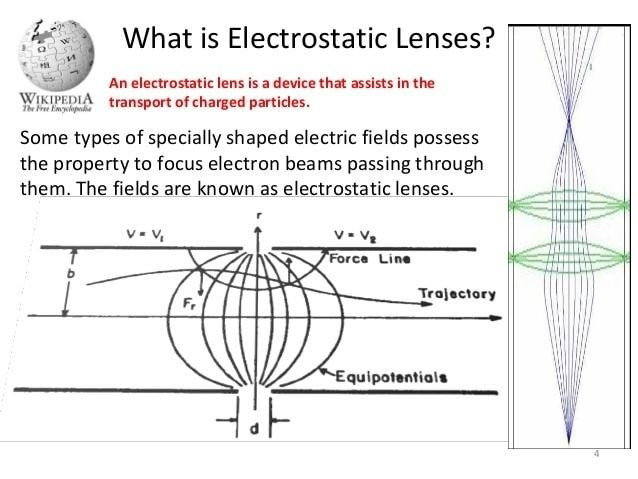## Experiment 6 electrostatic lens

An electrostatic lens is a device that assists in the transport of charged particles. For instance, it can guide electrons emitted from a sample to an electron analyzer, analogous to the way an optical lens assists in the transport of light in an optical instrument. Systems of electrostatic lenses can be designed in the same way as optical lenses, so electrostatic lenses easily magnify or converge the electron trajectories. An electrostatic lens can also be used to focus an ion beam, for example to make a microbeam for irradiating individual cells.

## Contents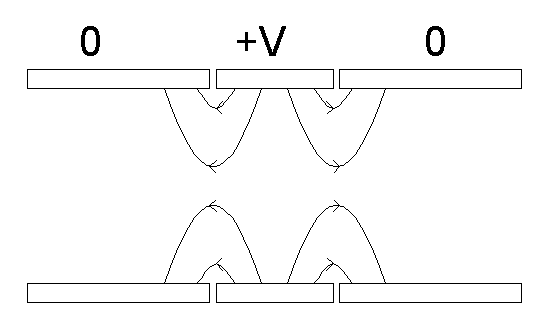## Cylinder lens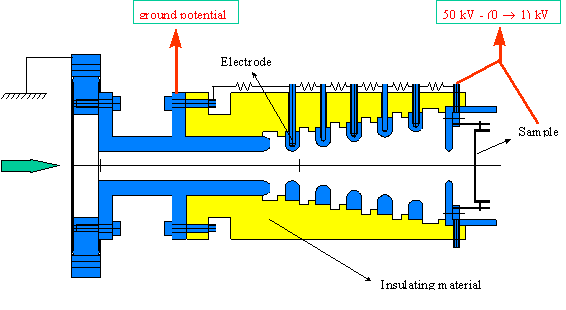A cylinder lens consists of several cylinders whose sides are thin walls. Each cylinder lines up parallel to the optical axis into which electrons enter. There are small gaps put between the cylinders. When each cylinder has a different voltage, the gap between the cylinders works as a lens. The magnification is able to be changed by choosing different voltage combinations. Although the magnification of two cylinder lenses can be changed, the focal point is also changed by this operation. Three cylinder lenses achieve the change of the magnification while holding the object and image positions because there are two gaps that work as lenses. Although the voltages have to change depending on the electron kinetic energy, the voltage ratio is kept constant when the optical parameters are not changed.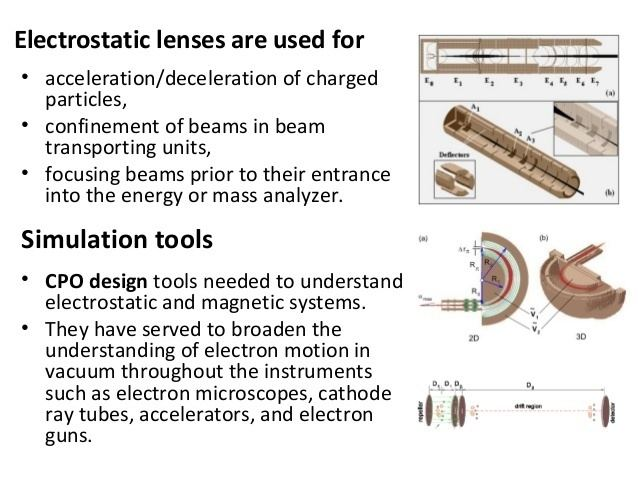While a charged particle is in an electric field force acts upon it. The faster the particle the smaller the accumulated impulse. For a collimated beam the focal length is given as the initial impulse divided by the accumulated (perpendicular) impulse by the lens. This makes the focal length of a single lens a function of the second order of the speed of the charged particle. Single lenses as known from photonics are not easily available for electrons.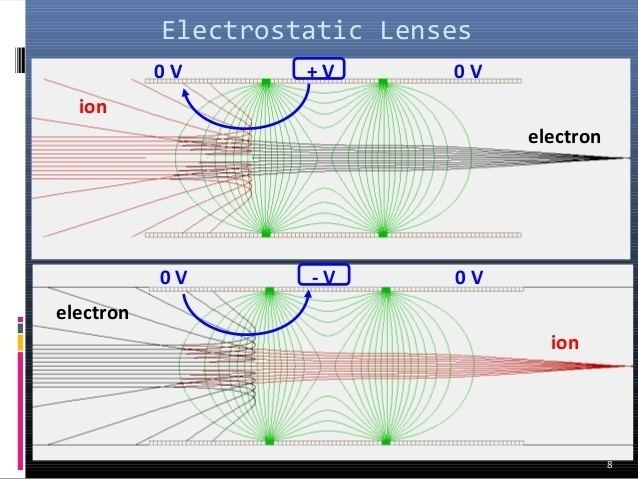The cylinder lens consists of defocusing lens, a focusing lens and a second defocusing lens, with the sum of their refractive powers being zero. But because there is some distance between the lenses, the electron makes three turns and hits the focusing lens at a position farther away from the axis and so travels through a field with greater strength. This indirectness leads to the fact that the resulting refractive power is the square of the refractive power of a single lens.

## Einzel lens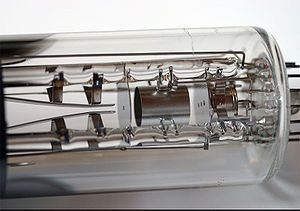An einzel lens is an electrostatic lens that focuses without changing the energy of the beam. It consists of three or more sets of cylindrical or rectangular tubes in series along an axis.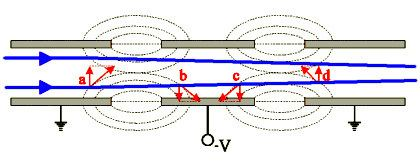The quadrupole lens consists of two single quadrupoles turned 90° with respect to each other. Let z be the optical axis then one can deduce separately for the x and the y axis that the refractive power is again the square of the refractive power of a single lens.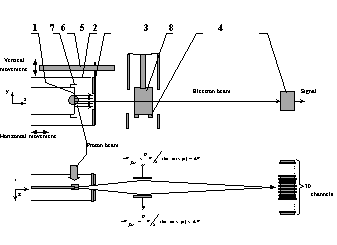A magnetic quadrupole works very similar to an electric quadrupole. But the Lorentz force increases with the velocity of the charged particle. In spirit of a Wien filter a combined magnetic, electric quadrupole is achromatic around a given velocity. Bohr and Pauli claim that this lens leads to aberration when applied to ions with spin (in the sense of chromatic aberration), but not when applied to electrons, which also have a spin. See Stern–Gerlach experiment.

## Magnetic lens

Instead of an electrostatic field we can also use a magnetic field to focus charged particles. The Lorentz force acting on the electron is perpendicular to both the direction of motion, and to the direction of the magnetic field (vxB). A homogeneous field deflects charged particles, but does not focus them. The simplest magnetic lens is a donut-shaped coil through which the beam passes, preferably along the axis of the coil. To generate the magnetic field an electric current is passed through the coil. The magnetic field is strongest in the plane of the coil, and gets weaker as we move away from it. In the plane of the coil, the field gets stronger as we move away from the axis. Thus, a charged particle further from the axis experiences a stronger Lorentz force than a particle closer to the axis (assuming that they have the same velocity). This gives rise to the focusing action. Unlike the paths in an electrostatic lens, the paths in a magnetic lens contain a spiraling component, i.e. the charged particles spiral around the optical axis. As a consequence, the image formed by a magnetic lens is rotated relative to the object. This rotation is absent for an electrostatic lens. The spatial extent of the magnetic field can be controlled by using an iron (or other magnetically soft material) magnetic circuit. This makes it possible to design and build more compact magnetic lenses with well defined optical properties. The vast majority of electron microscopes in use today use magnetic lenses due to their superior imaging properties, and the absence of the high voltages that are required for electrostatic lenses.

## Multipole lenses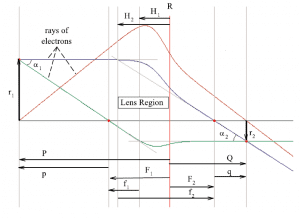Multipoles beyond the quadrupole can correct for spherical aberration and in particle accelerators the dipole bending magnets are really composed of a large number of elements with different superpositions of multipoles.

Usually the dependency is given for the kinetic energy itself depending on the power of the velocity. So for an electrostatic lens the focal length varies with the second power of the kinetic energy, while for a magnetostatic lens the focal length varies proportional to the kinetic energy. And a combined quadrupole can be achromatic around a given energy.

If a distribution of particles with different kinetic energies is accelerated by a longitudinal electric field, the relative energy spread is reduced leading to less chromatic error for example in the electron microscope.

## Electron spectroscopy

The recent development of electron spectroscopy makes it possible to reveal the electronic structures of molecules. Although this is mainly accomplished by electron analysers, electrostatic lenses also play a significant role in the development of electron spectroscopy.

Since electron spectroscopy detects several physical phenomena from the electrons emitted from samples, it is necessary to transport the electrons to the electron analyser. Electrostatic lenses satisfy the general properties of lenses.

## References

Similar Topics
Halloween (1978 film)